# Creating Calculated Measures

## Have your Indicators and Performance Measures automatically calculate their data values

With Clear Impact Scorecard, you can set your Indicators and Performance Measures automatically calculate values by combining data points from other Indicators and Performance Measures. For the sake of this article we will refer to both Indicators and Performance Measures as "Measures" since the process for both of them is the same.

Some important things to know:

• Once a measure has a calculation type set, Actual Values cannot be entered manually and must be calculated using data values from other measures.
• Changing a measure to Calculated once it already has Actual or Target Values entered will delete all the existing values.
• Calculated measures can be "nested" within one another so you can roll up data from city, to county, to region, to national by using several levels of calculated measures.
• Calculated measures appear throughout the scorecard with a green or red circle on the left side of the Indicator or Performance Measure icon. The circle is green if all data values are reporting and red if some disaggregated data values are missing.## Calculated Measure Best Practices

1. Same Reporting Frequency - When creating calculated measures, the same reporting frequency is necessary for all measures involved.

2. Reliable Personnel- Make sure the people and organizations responsible for inputting the data at each level will input reliable data; otherwise, your calculated measures will not have accurate, updated or useful data.

3. Standard Measure Names - If you are "rolling up" measures from many levels such as city, county or state, be sure to use standard naming and / or settings scheme for the measures. This will ensure that they are all identical except for the tag, making easier to find and manage them all.

4. Plan on Paper First - Planning out a complex roll up of measures on paper first is a wise idea! This method holds the abstract nature of the process together and helps users visualize the data roll up while it is being built and used so sections of measures are not forgotten.

5. Select Measures Carefully - When doing large scale data entry, it is important to remember that Clear Impact Scorecard is a strategy management system and is not intended for managing hundreds of measures in a single calculation chain as this will slow down performance.

When building many measures, ask yourself: Will we ever fill out the turn the curve page for this measure? If not, then the measure does not provide much value in Clear Impact Scorecard. The software is designed for conversations on how to turn the curve and what action steps to take.

## Creating a Calculated Measure

To create a calculated measure, follow the steps in the Indicator or Performance Measure support pages to learn how to create a new measure.

Edit the general properties on the Indicator or Performance Measure page then change the Calculation Type to a specific calculation.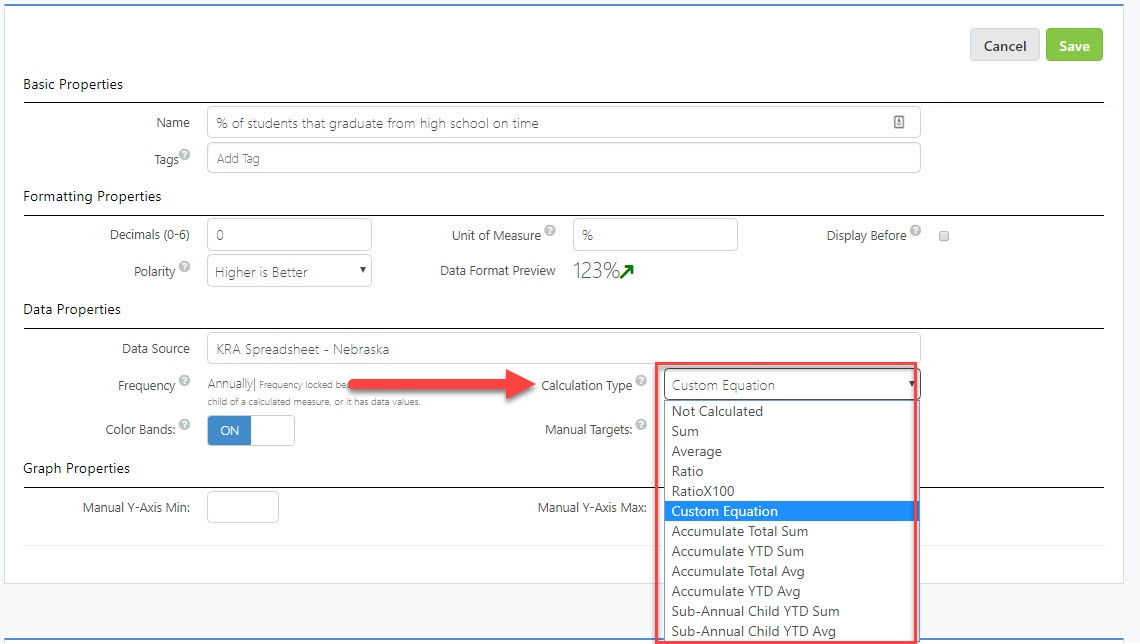Once you have selected a Calculation Type, save the measure and edit the data values section to configure the calculation.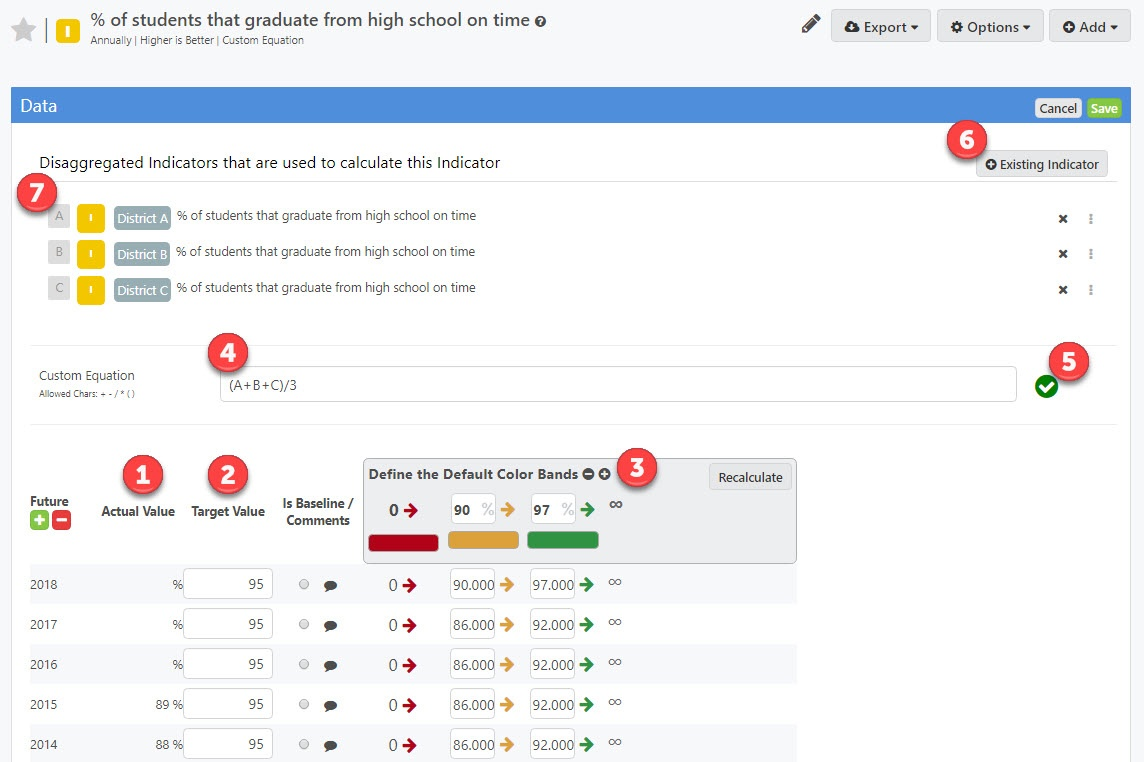1. The Actual Value column displays the end product of the calculation on the disaggregated measures Actual Values.

2. The Target Value column displays the end product of the calculation on the disaggregated measures Target Values.

3. Color bands can be configured normally just like any other measure. The color bands only appear if a Target Value can be calculated for that time period. The Recalculate button will automatically configure any new percentages entered into the Color Bands defaults.

4. The custom equation bar appears when using the custom equation calculated measure type. For more information on this check out the support article here.

5. The custom equation validation box will turn green if the formula is valid and be red if your formula is invalid.

7. When a measure is set to Calculated, the Disaggregated Measures section will appear in the data values section. This section displays the measures that are used in the calculation, any measure that appears in this list will have its data combined into the end value for the calculated measure. You can re-arrange the sort order or remove measures by using the grab icon on the right side. Some calculation types require the sort order to be a certain way for the calculation to run properly, see the Ratio calculation type below. You can remove measures by clicking on the X.

## Calculated Measure Types

Sum

The sum calculation type adds each disaggregated measures's data values for each time period and reports the total.

After the calculation is created, whenever the measures's Actual Values are entered for any time period, the calculated measure will reflect a calculation per the new values.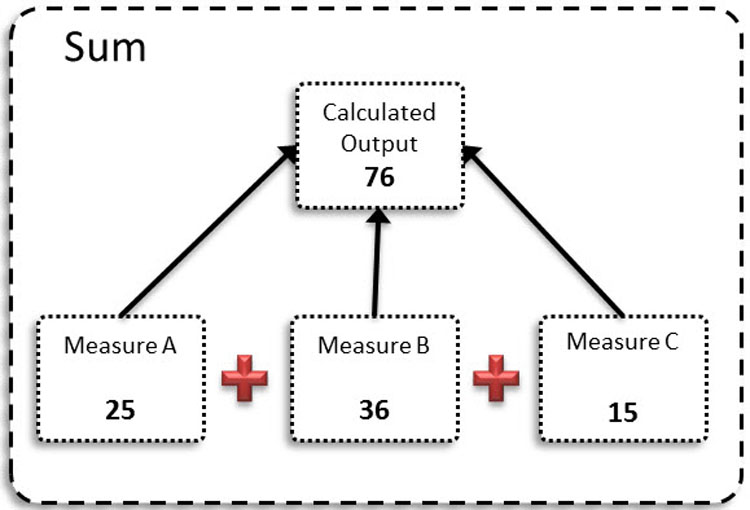## Average

The average calculation type takes an average of each disaggregated measure for each time period.

If you have 3 disaggregated measures being calculated and you enter data values for all 3, the calculation will run and divide by 3.

However, if you have 3 disaggregated measures and you only enter data values for 1 or 2, the measures will the calculation will run and only divide by 1 or 2 so your average will be accurate.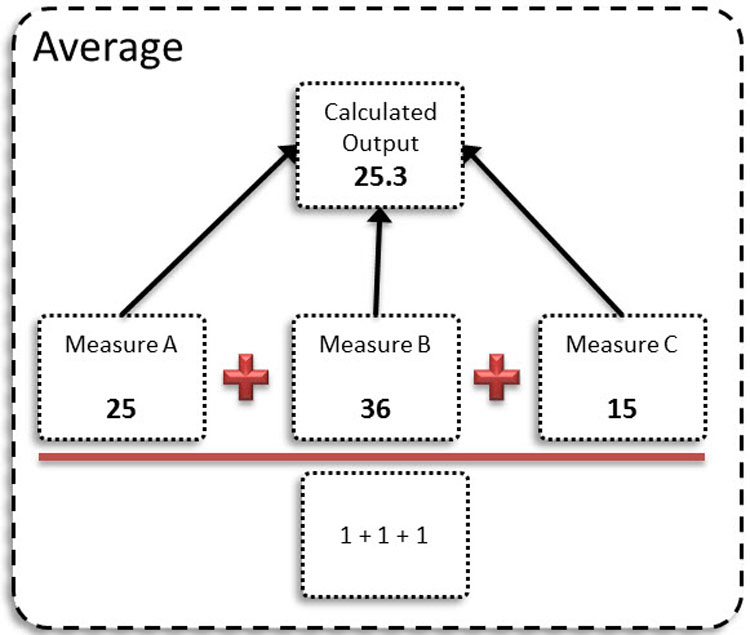## Ratio

The ratio calculation type takes a ratio of two disaggregated measures.

The measure that appears first in the list of disaggregated measures will be the numerator and the measure that appears second will be the denominator.

To change the order of these measures, simply use the drag and drop handles on the right side.

Note: Having more than two disaggregated measures with this calculation will not run properly.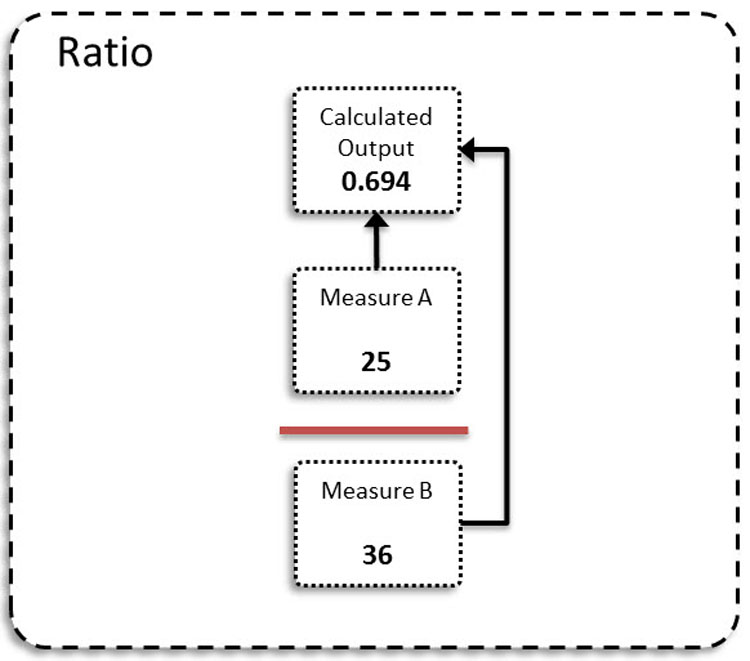## Ratio x 100

This calculation type behaves the same way as the Ratio type above except the output is multiplied by 100.